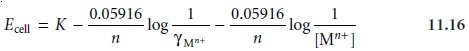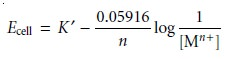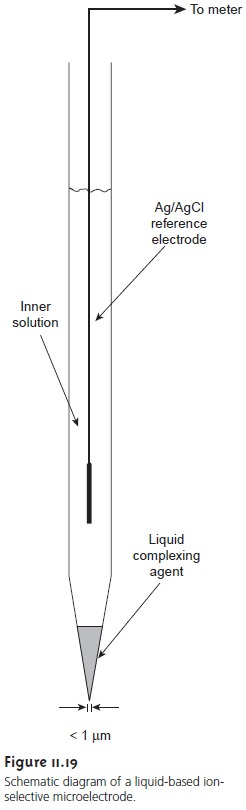Home | | Modern Analytical Chemistry | Quantitative Applications - Potentiometric Methods of Analysis

# Quantitative Applications - Potentiometric Methods of AnalysisThe potentiometric determination of an analyte’s concentration is one of the most common quantitative analytical techniques.

Quantitative Applications

The potentiometric determination of an analyte’s concentration is one of the most common quantitative analytical techniques. Perhaps the most frequently employed, routine quantitative measurement is the potentiometric determination of a solu- tion’s pH, a technique considered in more detail in the following discussion. Other areas in which potentiometric applications are important include clinical chemistry, environmental chemistry, and potentiometric titrations. Before considering these applications, however, we must first examine more closely the relationship between cell potential and the analyte’s concentration, as well as methods for standardizing potentiometric measurements.

## Activity Versus Concentration

In describing metallic and membrane indicator elec- trodes, the Nernst equation relates the measured cell potential to the concentration of analyte. In writing the Nernst equation, we often ignore an important detail—the potential of an electrochemical cell is a function of activity, not concen- tration. Thus, the Nernst equation for a metallic electrode of the first kind is more appropriately written aswhere aMn+ is the activity of the metal ion. As described, the activity of an ion is equal to the product of its concentration, [Mn+], and a matrix-dependent activity coefficient, γMn+.Substituting equation 11.15 into equation 11.14 and rearranging givesEquation 11.16 can be solved for the metal ion’s concentration if its ac- tivity coefficient is known. This presents a serious complication since the activity coefficient may be difficult to determine. If, however, the standards and samples have an identical matrix, then γMn+ remains constant, and equation 11.16 simplifies towhere K’ includes the activity coefficient.

## Quantitative Analysis Using External Standards

To determine the concentration of analyte in a sample, it is necessary to standardize the electrode. If the electrode’s response obeys the Nernst equation, then only the constant K need be determined, and standardizing with a single ex- ternal standard is possible. Since small deviations from the ideal “Nerstian” slope of ±RT/nF or ±RT/zF are frequently observed, standardization is usually accom- plished using two or more external standards.

In most quantitative analyses we are interested in determining the concen- tration, not the activity, of the analyte. As noted earlier, however, the electrode’s response is a function of the analyte’s activity. In the absence of interferents, a calibration curve of potential versus activity is a straight line. A plot of poten- tial versus concentration, however, may be curved at higher concentrations of analyte due to changes in the analyte’s activity coefficient. A curved calibra- tion curve may still be used to determine the analyte’s concentration if the stan- dard’s matrix matches that of the sample. When the exact composition of the sample matrix is unknown, which often is the case, matrix matching becomes impossible.

Another approach to matrix matching, which does not rely on knowing the exact composition of the sample’s matrix, is to add a high concentration of inert electrolyte to all samples and standards. If the concentration of added electrolyte is sufficient, any difference between the sample’s matrix and that of the standards becomes trivial, and the activity coefficient remains essentially constant. The solution of inert electrolyte added to the sample and standards is called a total ionic strength adjustment buffer (TISAB).## Quantitative Analysis Using the Method of Standard Additions

Because of the dif- ficulty of maintaining a constant matrix for samples and standards, many quantita- tive potentiometric methods use the method of standard additions. A sample of vol- ume, VX, and analyte concentration, CX, is transferred to a sample cell, and the potential, (Ecell)X, measured. A standard addition is made by adding a small volume, VS, of a standard containing a known concentration of analyte, CS, to the sample, and the potential, (Ecell)S, measured. Provided that VS is significantly smaller than VX, the change in sample matrix is ignored, and the analyte’s activity coefficient re- mains constant. Example 11.7 shows how a one-point standard addition can be used to determine the concentration of an analyte.## Free Ions Versus Complexed Ions

In discussing the F ion-selective electrode, we noted that the membrane potential is influenced by the concentration of F, but not the concentration of HF. An analysis for fluoride, therefore, is pH-dependent. Below a pH of approximately 4, fluoride is present predominantly as HF, and a quantitative analysis for total fluoride is impossible. If the pH is increased to greater than 4, however, the equilibrium

HF(aq)+ H2O(l) < = = = = > H3O+(aq)+ F(aq)

shifts to the right, and a quantitative analysis for total fluoride is possible.

Most potentiometric electrodes are selective for only the free, uncomplexed analyte and do not respond to complexed forms of the analyte. Solution condi- tions, therefore, must be carefully controlled if the purpose of the analysis is to de- termine the analyte’s total concentration. On the other hand, this selectivity pro- vides a significant advantage over other quantitative methods of analysis when it is necessary to determine the concentration of free ions. For example, calcium is present in urine both as free Ca2+ ions and as protein-bound Ca2+ ions. If a urine sample is analyzed by atomic absorption spectroscopy, the signal is proportional to the total concentration of Ca2+, since both free and bound calcium are atomized. Analysis with a Ca2+ ISE, however, gives a signal that is a function of only free Ca2+ ions since the protein-bound ions cannot interact with the electrode’s membrane.

## Representative Method

Ion-selective electrodes find application in numerous quantitative analyses,  each of  which  has  its own unique considerations. The following pro- cedure for the analysis of fluoride in toothpaste provides an instructive example.

## Measurement of pH

With the availability of inexpensive glass pH electrodes and pH meters, the determination of pH has become one of the most frequent quantita- tive analytical measurements. The potentiometric determination of pH, however, is not without complications, several of which are discussed in this section.

One complication is the meaning of pH. The conventional definition of pH as presented in most introductory texts is

pH = –log [H+]       ………..11.17

The pH of a solution, however, is defined by the response of an electrode to the H+ ion and, therefore, is a measure of its activity.

pH = –log(aH+)      ………..11.18

Calculating the pH of a solution using equation 11.17 only approximates the true pH. Thus, a solution of 0.1 M HCl has a calculated pH of 1.00 using equation 11.17, but an actual pH of 1.1 as defined by equation 11.18.8 The difference between the two values occurs because the activity coefficient for H+ is not unity in a matrix of 0.1 M HCl. Obviously the true pH of a solution is affected by the composition of its matrix. As an extreme example, the pH of 0.01 M HCl in 5 m LiCl is 0.8, a value that is more acidic than that of 0.1 M HCl!

A second complication in measuring pH results from uncertainties in the rela- tionship between potential and activity. For a glass membrane electrode, the cell po- tential, EX, for a solution of unknown pH is given aswhere K includes the potential of the reference electrode, the asymmetry potential of the glass membrane and any liquid junction potentials in the electrochemical cell. All the contributions to K are subject to uncertainty and may change from day to day, as well as between electrodes. For this reason a pH electrode must be calibrated using a standard buffer of known pH. The cell potential for the standard, ES, iswhere pHS is the pH of the standard. Subtracting equation 11.20 from equation 11.19 and solving for pH giveswhich is the operational definition of pH adopted by the International Union of Pure and Applied Chemistry.*

Calibrating the electrode presents a third complication since a standard with an accurately known activity for H+ needs to be used. Unfortunately, it is not possible to calculate rigorously the activity of a single ion. For this reason pH electrodes are calibrated using a standard buffer whose composition is chosen such that the de- fined pH is as close as possible to that given by equation 11.18. Table 11.6 gives pH values for several primary standard buffer solutions accepted by the National Insti- tute of Standards and Technology.A pH electrode is normally standardized using two buffers: one near a pH of 7 and one that is more acidic or basic depending on the sample’s expected pH. The pH electrode is immersed in the first buffer, and the “standardize” or “calibrate” control is adjusted until the meter reads the correct pH. The electrode is placed in the second buffer, and the “slope” or “temperature” control is adjusted to the- buffer’s pH. Some pH meters are equipped with a temperature compensation fea- ture, allowing the pH meter to correct the measured pH for any change in tempera- ture. In this case a thermistor is placed in the sample and connected to the pH meter. The “temperature” control is set to the solution’s temperature, and the pH meter is calibrated using the “calibrate” and “slope” controls. If a change in the sample’s temperature is indicated by the thermistor, the pH meter adjusts the slope of the calibration based on an assumed Nerstian response of 2.303RT/F.

## Clinical Applications

Perhaps the area in which ion-selective electrodes receive the widest use is in clinical analysis, where their selectivity for the analyte in a complex matrix provides a significant advantage over many other analytical methods. The most common analytes are electrolytes, such as Na+, K+, Ca2+, H+, and Cl, and dis- solved gases, such as CO2. For extracellular fluids, such as blood and urine, the analy- sis can be made in vitro with conventional electrodes, provided that sufficient sample is available. Some clinical analyzers place a series of ion-selective electrodes in a flow cell, allowing several analytes to be monitored simultaneously.

Standards, samples, and rinse solutions are pumped through the flow cell and across the surface of the electrodes. For smaller volumes of sample the analysis can be conducted using dis- posable ion-selective systems, such as the Kodak Ektachem analyzer for K+ shown in Figure 11.18. The analyzer consists of separate electrodes for the sample and refer- ence solutions. Each electrode is constructed from several thin films, consisting of a Ag/AgCl reference electrode, a salt bridge and an ion-selective membrane, deposited on a support base. The two electrodes are connected by a paper salt bridge saturated with the sample and reference solutions. The overall dimensions of the analyzer are 2.8 cm x 2.4 cm with a thickness of 150 μm  and require only 10 μL each of sample and reference solution. Similar analyzers are available for the determination of Na+, Cl, and CO2.The analysis of intercellular fluids requires an ion-selective electrode that can be inserted directly into the desired cell. Liquid-based membrane microelec- trodes with tip diameters of less than 1 μm are constructed by heating and draw- ing out a hard-glass capillary tube with an initial diameter of approximately 1–2 mm (Figure 11.19). The tip of the microelectrode is made hydrophobic by dipping in dichlorodimethyl silane. An inner solution appropriate for the desired analyte and a Ag/AgCl wire reference electrode are placed within the microelec- trode. The tip of the microelectrode is then dipped into a solution containing the liquid complexing agent. The small volume of liquid complexing agent entering the microelectrode is retained within the tip by capillary action, eliminating the need for a solid membrane. Potentiometric microelectrodes have been developed for a number of clinically important analytes, including H+, K+, Na+, Ca2+, Cl, and I.

## Environmental Applications

Although ion-selective electrodes find use in envi- ronmental analysis, their application is not as widespread as in clinical analysis. Standard methods have been developed for the analysis of CN, F, NH3, and NO3in water and wastewater. Except for F, however, other analytical methods are con- sidered superior. By incorporating the ion-selective electrode into a flow cell, the continuous monitoring of wastewater streams and other flow systems is possible. Such applications are limited, however, by the electrode’s response to the analyte’s activity, rather than its concentration. Considerable interest has been shown in the development of biosensors for the field screening and monitoring of environmental samples for a number of priority pollutants.

 3

## Potentiometric Titrations

We noted that one method for determining the equivalence point of an acid–base titration is to follow the change in pH with a pH electrode. The potentiometric determination of equivalence points is feasible for acid–base, complexation, redox, and precipitation titrations, as well as for titrations in aqueous and nonaqueous solvents. Acid–base, complexation, and precipitation potentiometric titrations are usually monitored with an ion-selective electrode that is selective for the analyte, although an electrode that is selective for the titrant or a reaction product also can be used. A redox electrode, such as a Pt wire, and a refer- ence electrode are used for potentiometric redox titrations.

Study Material, Lecturing Notes, Assignment, Reference, Wiki description explanation, brief detail
Modern Analytical Chemistry: Electrochemical Methods of Analysis : Quantitative Applications - Potentiometric Methods of Analysis |